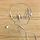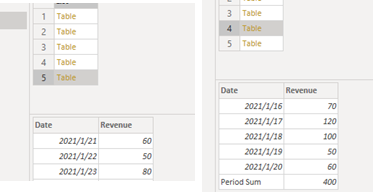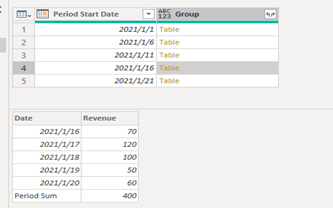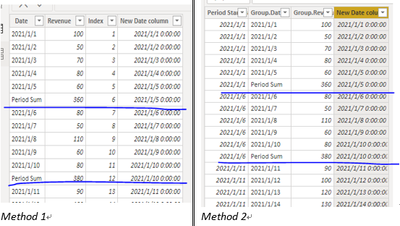cancel
Showing results for
Did you mean:

Fabric is Generally Available. Browse Fabric Presentations. Work towards your Fabric certification with the Cloud Skills Challenge.## Power Query: Insert Sum Rows for Dynamic Periods

Sample Table

In the following scenario, I would like to insert a sum row for each 5 days as an example.Method 1

``````let
Source = Table.FromRows(Json.Document(Binary.Decompress(Binary.FromText("bdBLCsAgDATQu7iWkon1dxbx/tdoAqWQjrh7yiTjWklFcdlJOUEk7fyRGtUgxaQHuU1GkGrSgjR60yl5+HgEmhRk9/8k+N4zknIV+ObQaL47SrTKExqVRj+kjcPUST1VqJWCib9ey7vZfgA=", BinaryEncoding.Base64), Compression.Deflate)), let _t = ((type nullable text) meta [Serialized.Text = true]) in type table [Date = _t, Revenue = _t]),
#"Changed Type" = Table.TransformColumnTypes(Source,{{"Date", type date}, {"Revenue", Int64.Type}}),
#"Grouped Rows" = List.Transform({0..Number.RoundDown(Table.RowCount(#"Changed Type")/5)},each [A=Table.Range(#"Changed Type",_*5,5),B=A&#table({"Date","Revenue"},{{"Period Sum",List.Sum(A[Revenue])}})][B]),
#"Combine Tables" = Table.Combine(#"Grouped Rows")
in
#"Combine Tables"
``````

To separate the 23 days table with 5 days in each period, we will have 5 grouped tables which have 5 days in the first 4 tables, remaining with 3 days in the last table. To achieve the expected output, we can first Round Down the number of table to make the dates separate in to sub-tables. The next step is to add a sum row in each table, we can just use #table to build a single line table and connect it to the original sub-table.Finally, we can just combine the tables using Table.Combine(). And I would add another index column because the data type of the date column is no longer Date Type, this index column allows us to sort the dates in a consecutive order when creating report visuals.

Method 2

``````let
Source = Table.FromRows(Json.Document(Binary.Decompress(Binary.FromText("bdBLCsAgDATQu7iWkon1dxbx/tdoAqWQjrh7yiTjWklFcdlJOUEk7fyRGtUgxaQHuU1GkGrSgjR60yl5+HgEmhRk9/8k+N4zknIV+ObQaL47SrTKExqVRj+kjcPUST1VqJWCib9ey7vZfgA=", BinaryEncoding.Base64), Compression.Deflate)), let _t = ((type nullable text) meta [Serialized.Text = true]) in type table [Date = _t, Revenue = _t]),
#"Changed Type" = Table.TransformColumnTypes(Source,{{"Date", type date}, {"Revenue", Int64.Type}}),
#"Group Table" = Table.Group( #"Changed Type","Date",{"Group",each _&#table({"Date","Revenue"},{{"Period Sum",List.Sum([Revenue])}})},0,(x,y)=>Number.From(y-x>=#duration(5,0,0,0))),
#"Renamed Columns" = Table.RenameColumns(#"Group Table",{{"Date", "Period Start Date"}}),
#"Expanded Group" = Table.ExpandTableColumn(#"Renamed Columns", "Group", {"Date", "Revenue"}, {"Group.Date", "Group.Revenue"})
in
#"Expanded Group"
``````

Here is another method, we can use Table.Group() to group dates in tables. Again we used #table to build a single line table(the sum row) and connect it to the subtables.

It is critical that the underlined argument “0” stands for GroupKind.Local, which means the previous Sum Revenue expression will only sum up the revenue in each table group. If I replace “0” with “1”, the context would be GroupKind.Global which means the List.Sum([Revenue]) will sum up the entire column and display the result in the last row. The last argument in the step separates the consecutive dates into groups every 5 days.I also renamed Date column to Period Start Date before expanding the tables, so I can use it to sort the dates in report visuals.

Extension
In addition, it is possible to bring query parameters into the solution. We just need to create a parameter and replace the underlined day argument in duration() expression in method 2 with the parameter name. so we can just change the parameter to set how many days we would like to have in each period.Conclusion

As can be seen, both solutions return the desired output. However, there is a downside that the date column is changed to Text type data, which means the date hierarchy is no longer available for any calculation related to calendar. Thus, for those users that still need date hierarchy, we could add another Date column in the table. We then need to connect new date column to the calendar date column with Many to One relationship, because we want the Period Sum date to be the last date in each period, which means there will be duplicated date value and the new date column is no longer unique.

``````Method 1 New Date = IF([Date]="Period Sum",CALCULATE(MAX([Date]),FILTER('Table',[Index]=EARLIER('Table'[Index])-1)),[Date])

Method 2 New Date = IF([Group.Date]="Period Sum",CALCULATE(MIN([Period Start Date]),FILTER('Table (2)',[Period Start Date]>EARLIER([Period Start Date])))-1,DATEVALUE([Group.Date]))
``````Author: Paul Zheng

Reviewer: Ula Huang, Kerry Wang

Top Kudoed Posts
Latest Articles